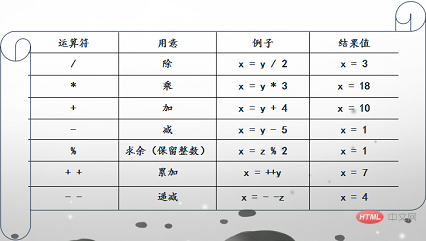# JavaScript算数运算符```算数运算符 (算术运算符用于执行变量与/或值之间的算术运算)
加号+(+ 运算符用于把文本值或字符串变量加起来（连接起来）)
var x=5,y=6
document.write("5+6="+(x+y)+"<br>")
//减号——
document.write("5-6="+(x-y)+"<br>")
// 乘*
document.write("5*6="+(x*y)+"<br>")
// 除 /
document.write("5/6="+(x/y)+"<br>")
// 求余数 %
document.write("5%6="+(x%y)+"<br>")
// 累加++
document.write("++5="+(++x)+"<br>")
// 递减--
document.write("--6="+(--y)+"<br>")```

### 任务

?不会了怎么办

php中文网
phpstudy

QQ交流群